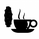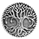5889 visningar
The Sharpe ratio allows you to see whether or not an investment has historically provided a return appropriate to its risk level. A Sharpe ratio above one is acceptable, above 2 is good, and above 3 is excellent. A Sharpe ratio less than one would indicate that an investment has not returned a high enough return to justify the risk of holding it. Interesting in this example, SPY's one year avg Sharpe ratio is above 3. This would mean on average SPY returns 3x better returns than the risk associated with holding it, implying there is some sort of underlying value to the investment.

When the sharpe ratio is above its signal, this implies the investment is currently outperforming compared to its typical return, below the signal means the investment is currently under performing. A negative Shape would mean that the investment has not provided a positive return, and may be a possible short candidate.
Open-source script

In true TradingView spirit, the author of this script has published it open-source, so traders can understand and verify it. Cheers to the author! You may use it for free, but reuse of this code in a publication is governed by House Rules. You can favorite it to use it on a chart.

Want to use this script on a chart?
```study("Trailing Sharpe Ratio")
src = ohlc4, len = input(252, title = "Time Frame (252 is one year)")
//mean = sma(src,len)
dividend_yield = input(0.0000, minval = 0.00001, title = "Dividend Yield? Enter as Decimal, USE 12 MONTH TTM!!!")
pc = ((src - src[len])/src) + (dividend_yield*(len/252))
std = stdev(src,len)
stdaspercent = std/src
riskfreerate = input(0.0004, minval = 0.0001, title = "risk free rate (3 month treasury yield), enter as decimal")
sharpe = (pc - riskfreerate)/stdaspercent
signal = sma(sharpe,len)
calc = sharpe - signal
plot(sharpe, style = line, color = calc < 0 ? red : green, linewidth = 2)
plot(signal, style = line, color = purple, linewidth = 2)```

## KommentarerDefinitely one of my favorite scripts, would like to see it turned into a strategy.
SvaraKoko0111855
@Koko0111855, how did you solve an error on a line that does not exist?
Svara